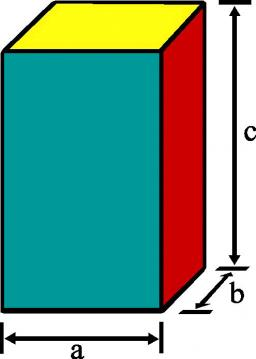# Volume and body diagonal

Calculate how much the volume and body diagonal of the cuboid decrease if we reduce each of its three edges a, b, c by 18%?

Correct result:

p1 =  44.8632 %
p2 =  18 %

#### Solution:

${p}_{2}=p=18=18\mathrm{%}$We would be pleased if you find an error in the word problem, spelling mistakes, or inaccuracies and send it to us. Thank you!Tips to related online calculatorsThe cube-shaped box is filled to the brim with 2 liters of milk. Calculate the edge and surface of the box.